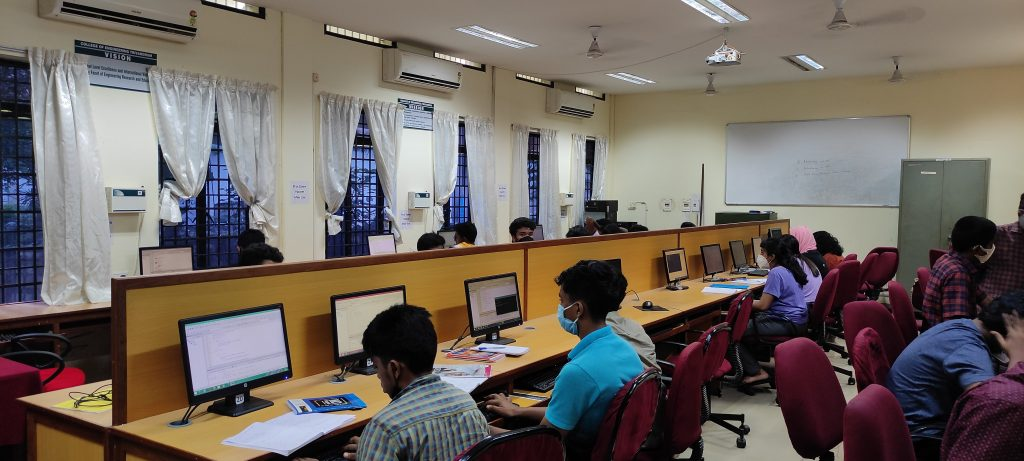# Computer LabLab in charge:

• Faculty: Dr. Sanil K Danial
• Technical Staff: Mr. Sreekanth K

Details of Labs conducted:

• ECL201 – SCIENTIFIC COMPUTING LAB – S3 AEI, ECE
• AE431 – CONTROL SYSTEM AND SIGNAL PROCESSING LAB – S7 AEI
• 01EC6293 – COMMUNICATION SYSTEM LAB – M1 MTV
• 01EC6393 – DSP SYSTEMS LAB – M1 SP
• 01EC6394 – IMAGE PROCESSING LAB – M2 SP

### ECL201 – SCIENTIFIC COMPUTING LAB

The following experiments are designed to translate the mathematical concepts into system design.

• The students shall use Python for realization of experiments. Other softwares such as R/MATLAB/SCILAB/LabVIEW can also be used.
• The experiments will lay the foundation for future labs such as DSP lab.
• The first two experiments are mandatory and any six of the rest should be done.”

LIST OF EXPERIMENTS:

1 Familarization of the Computing Tool
2 Familarization of Scientific Computing
3 Realization of Arrays and Matrices
4 Numerical Differentiation and Integration
5 Solution of Ordinary Differential Equations
6 Simple Data Visualization
7 Simple Data Analysis with Spreadsheets
8 Convergence of Fourier Series
9 Coin Toss and the Level Crossing Problem

### AE431 – CONTROL SYSTEM AND SIGNAL PROCESSING LAB

This course aims to:

• To give hands on experience in various digital Signal Processing techniques using TMS 320C6X family processors and in control system analysis using MATLAB.
• At the end of the semester students are expected to be familiar with the basic s ignal processing & control system techniques.

LIST OF EXPERIMENTS:

CONTROL SYSTEM LAB using MATLAB:

1 Familiarization of MATLAB commands used in control system design
2 Representation of system in MATLAB: state space representation & tr ansfer function representation
3 Stability analysis using Bode plot, root locus & their pole pole-zerozero-gain representation.
4 Implementation of Ziegler Ziegler- Nicholas/ Cohen Cohen-coon tuning method for 1st order system.
5 Analysis of a closed loop system.
6 Implementation of PID control using both m m-file and Simulink.
7 Pole placement technique applied to stabilize a system.
8 Realization of a compensator design.
9 Modelling and analysis of a first order system.
10 Modelling of an unstable system (inverted pendulum, ball & plate system etc.)

PC Based Control:

1 PLC programming: familiarization of instruction set.
2 PLC programming: simulation of process control.
4 Familiarization of Distributed Control System (DCS) with different process stations press ure, flow and level.

LabVIEW based Virtual Instrumentation:

1 Getting started with LabVIEW: Basic operations, controls, indicators, and simple Programming structures.
2 Debugging a VI and sub sub-VI.
3 Familiarization of DAQ card.

SIGNAL PROCESSING LAB:

1 Familiarization of signal processing commands used in MATLAB Software.
2 Developing elementary signal function modules (m-files) for unit impulse, step, exponent and ramp sequence.
3 Generating continuous and discrete time sequences.
4 Carrying out mathematical operations on signals.
5 Response of LTI system described by difference and differential equation.
6 Developing a program for computing inverse Z-Transform.
7 Developing program for finding magnitude & phase response of LTI System
8 Developing program for computing DFT & IDFT.
9 Developing a program for computing circular convolution.
10 Design of filter: FIR, IIR, ECG Signal filter (can be done as 3 separate experiments).

### 01EC6293 – COMMUNICATION SYSTEM LAB

This course aims to:

• Attain ability to do projects for digital communication
• Familiarize the use of MATLAB for simulation of optical communication
• Familiarize with microwave bench setup and measurements.

LIST OF EXPERIMENTS:

1 Simulation of Digital communication system
2 Simulation of fading and multipath channels
3 BER curves and eye patterns
4 Simulation of a RADAR System
5 Simulation of Mobile Network

### 01EC6393 – DSP SYSTEMS LAB

This course aims to:

• Attain ability to develop projects using DSP processors
• Familiarize the use of DSP processor based system for real time applications
• Develop skill to use higher level as well as assembly language for implementation of DSP based system

LIST OF EXPERIMENTS:

1. Development Environment
2. High Level Language Project Development
3. Assembly Optimizations
4. Memory Map
5. Real Time Processing.
6. Mini Project (Compulsory)

### 01EC6394 – IMAGE PROCESSING LAB

This course aims to:

• Implement the various image processing algorithms in MATLAB/C/C++.

LIST OF EXPERIMENTS:

1. Representation of Grayscale and colour images
2. Image transformations
3. Image Transforms
4. Image filtering
5. Edge detection
6. Segmentation
7. Morphological operation
8. Computed Tomography